Circle

"360 degrees" redirects here. For other uses, see 360 degrees (disambiguation).

Circle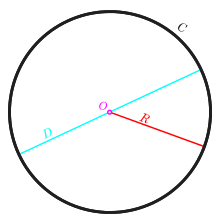A circle (black) which is measured by its circumference (C), diameter (D) in cyan, and radius (R) in red; its centre (O) is in magenta.

A circle is a simple closed shape in Euclidean geometry. It is the set of all points in a plane that are at a given distance from a given point, the centre; equivalently it is the curve traced out by a point that moves so that its distance from a given point is constant. The distance between any of the points and the centre is called the radius.

A circle is a simple closed curve which divides the plane into two regions: an interior and an exterior. In everyday use, the term "circle" may be used interchangeably to refer to either the boundary of the figure, or to the whole figure including its interior; in strict technical usage, the circle is only the boundary and the whole figure is called a disc.

A circle may also be defined as a special kind of ellipse in which the two foci are coincident and the eccentricity is 0, or the two-dimensional shape enclosing the most area per unit perimeter squared, using calculus of variations.

A circle is a plane figure bounded by one line, and such that all right lines drawn from a certain point within it to the bounding line, are equal. The bounding line is called its circumference and the point, its centre.
Euclid. Elements Book I.:4

Terminology

• Annulus: the ring-shaped object, the region bounded by two concentric circles.
• Arc: any connected part of the circle.
• Centre: the point equidistant from the points on the circle.
• Chord: a line segment whose endpoints lie on the circle.
• Circumference: the length of one circuit along the circle, or the distance around the circle.
• Diameter: a line segment whose endpoints lie on the circle and which passes through the centre; or the length of such a line segment, which is the largest distance between any two points on the circle. It is a special case of a chord, namely the longest chord, and it is twice the radius.
• Disc: the region of the plane bounded by a circle.
• Lens: the intersection of two discs.
• Passant: a coplanar straight line that does not touch the circle.
• Radius: a line segment joining the centre of the circle to any point on the circle itself; or the length of such a segment, which is half a diameter.
• Sector: a region bounded by two radii and an arc lying between the radii.
• Segment: a region, not containing the centre, bounded by a chord and an arc lying between the chord's endpoints.
• Secant: an extended chord, a coplanar straight line cutting the circle at two points.
• Semicircle: an arc that extends from one of a diameter's endpoints to the other. In non-technical common usage it may mean the diameter, arc, and its interior, a two dimensional region, that is technically called a half-disc. A half-disc is a special case of a segment, namely the largest one.
• Tangent: a coplanar straight line that touches the circle at a single point.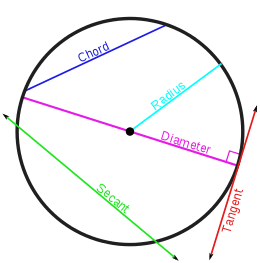Chord, secant, tangent, radius, and diameter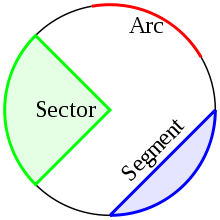Arc, sector, and segment

History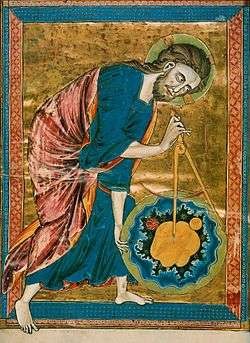The compass in this 13th-century manuscript is a symbol of God's act of Creation. Notice also the circular shape of the halo.

The word circle derives from the Greek κίρκος/κύκλος (kirkos/kuklos), itself a metathesis of the Homeric Greek κρίκος (krikos), meaning "hoop" or "ring". The origins of the words circus and circuit are closely related.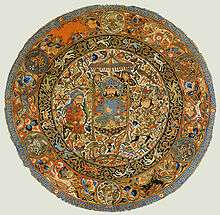Circular piece of silk with Mongol images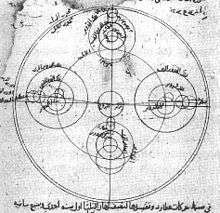Circles in an old Arabic astronomical drawing.

The circle has been known since before the beginning of recorded history. Natural circles would have been observed, such as the Moon, Sun, and a short plant stalk blowing in the wind on sand, which forms a circle shape in the sand. The circle is the basis for the wheel, which, with related inventions such as gears, makes much of modern machinery possible. In mathematics, the study of the circle has helped inspire the development of geometry, astronomy and calculus.

Early science, particularly geometry and astrology and astronomy, was connected to the divine for most medieval scholars, and many believed that there was something intrinsically "divine" or "perfect" that could be found in circles.

Some highlights in the history of the circle are:

• 1700 BCE – The Rhind papyrus gives a method to find the area of a circular field. The result corresponds to 256/81 (3.16049...) as an approximate value of π.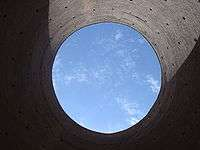Tughrul Tower from inside
• 300 BCE – Book 3 of Euclid's Elements deals with the properties of circles.
• In Plato's Seventh Letter there is a detailed definition and explanation of the circle. Plato explains the perfect circle, and how it is different from any drawing, words, definition or explanation.
• 1880 CE – Lindemann proves that π is transcendental, effectively settling the millennia-old problem of squaring the circle.

Analytic results

Length of circumference

Further information: Circumference

The ratio of a circle's circumference to its diameter is π (pi), an irrational constant approximately equal to 3.141592654. Thus the length of the circumference C is related to the radius r and diameter d by:Area enclosed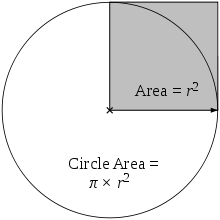Area enclosed by a circle = π × area of the shaded square
Main article: Area of a circle

As proved by Archimedes, in his Measurement of a Circle, the area enclosed by a circle is equal to that of a triangle whose base has the length of the circle's circumference and whose height equals the circle's radius, which comes to π multiplied by the radius squared:Equivalently, denoting diameter by d,that is, approximately 79% of the circumscribing square (whose side is of length d).

The circle is the plane curve enclosing the maximum area for a given arc length. This relates the circle to a problem in the calculus of variations, namely the isoperimetric inequality.

Equations

Cartesian coordinatesCircle of radius r = 1, centre (a, b) = (1.2, −0.5)

In an xy Cartesian coordinate system, the circle with centre coordinates (a, b) and radius r is the set of all points (x, y) such thatThis equation, known as the Equation of the Circle, follows from the Pythagorean theorem applied to any point on the circle: as shown in the adjacent diagram, the radius is the hypotenuse of a right-angled triangle whose other sides are of length |xa| and |yb|. If the circle is centred at the origin (0, 0), then the equation simplifies toThe equation can be written in parametric form using the trigonometric functions sine and cosine aswhere t is a parametric variable in the range 0 to 2π, interpreted geometrically as the angle that the ray from (a, b) to (x, y) makes with the positive x-axis.

An alternative parametrisation of the circle is:In this parametrisation, the ratio of t to r can be interpreted geometrically as the stereographic projection of the line passing through the centre parallel to the x-axis (see Tangent half-angle substitution). However, this parametrisation works only if t is made to range not only through all reals but also to a point at infinity; otherwise, the bottom-most point of the circle would be omitted.

In homogeneous coordinates each conic section with the equation of a circle has the formIt can be proven that a conic section is a circle exactly when it contains (when extended to the complex projective plane) the points I(1: i: 0) and J(1: i: 0). These points are called the circular points at infinity.

Polar coordinates

In polar coordinates the equation of a circle is:where a is the radius of the circle,is the polar coordinate of a generic point on the circle, andis the polar coordinate of the centre of the circle (i.e., r0 is the distance from the origin to the centre of the circle, and φ is the anticlockwise angle from the positive x-axis to the line connecting the origin to the centre of the circle). For a circle centred at the origin, i.e. r0 = 0, this reduces to simply r = a. When r0 = a, or when the origin lies on the circle, the equation becomesIn the general case, the equation can be solved for r, givingNote that without the ± sign, the equation would in some cases describe only half a circle.

Complex plane

In the complex plane, a circle with a centre at c and radius (r) has the equation. In parametric form this can be written.

The slightly generalised equationfor real p, q and complex g is sometimes called a generalised circle. This becomes the above equation for a circle with, since. Not all generalised circles are actually circles: a generalised circle is either a (true) circle or a line.

Tangent lines

The tangent line through a point P on the circle is perpendicular to the diameter passing through P. If P = (x1, y1) and the circle has centre (a, b) and radius r, then the tangent line is perpendicular to the line from (a, b) to (x1, y1), so it has the form (x1a)x + (y1b)y = c. Evaluating at (x1, y1) determines the value of c and the result is that the equation of the tangent isorIf y1b then the slope of this line isThis can also be found using implicit differentiation.

When the centre of the circle is at the origin then the equation of the tangent line becomesand its slope isProperties

Chord

• Chords are equidistant from the centre of a circle if and only if they are equal in length.
• The perpendicular bisector of a chord passes through the centre of a circle; equivalent statements stemming from the uniqueness of the perpendicular bisector are:
• A perpendicular line from the centre of a circle bisects the chord.
• The line segment through the centre bisecting a chord is perpendicular to the chord.
• If a central angle and an inscribed angle of a circle are subtended by the same chord and on the same side of the chord, then the central angle is twice the inscribed angle.
• If two angles are inscribed on the same chord and on the same side of the chord, then they are equal.
• If two angles are inscribed on the same chord and on opposite sides of the chord, then they are supplementary.
• An inscribed angle subtended by a diameter is a right angle (see Thales' theorem).
• The diameter is the longest chord of the circle.
• If the intersection of any two chords divides one chord into lengths a and b and divides the other chord into lengths c and d, then ab = cd.
• If the intersection of any two perpendicular chords divides one chord into lengths a and b and divides the other chord into lengths c and d, then a2 + b2 + c2 + d2 equals the square of the diameter.
• The sum of the squared lengths of any two chords intersecting at right angles at a given point is the same as that of any other two perpendicular chords intersecting at the same point, and is given by 8r 2 – 4p 2 (where r is the circle's radius and p is the distance from the centre point to the point of intersection).
• The distance from a point on the circle to a given chord times the diameter of the circle equals the product of the distances from the point to the ends of the chord.:p.71

Tangent

• A line drawn perpendicular to a radius through the end point of the radius lying on the circle is a tangent to the circle.
• A line drawn perpendicular to a tangent through the point of contact with a circle passes through the centre of the circle.
• Two tangents can always be drawn to a circle from any point outside the circle, and these tangents are equal in length.
• If a tangent at A and a tangent at B intersect at the exterior point P, then denoting the centre as O, the angles ∠BOA and ∠BPA are supplementary.
• If AD is tangent to the circle at A and if AQ is a chord of the circle, then DAQ = 1/2arc(AQ).

Theorems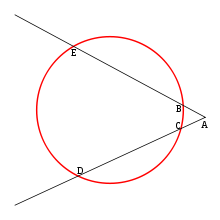Secant-secant theorem
• The chord theorem states that if two chords, CD and EB, intersect at A, then AC × AD = AB × AE.
• If two secants, AE and AD, also cut the circle at B and C respectively, then AC × AD = AB × AE. (Corollary of the chord theorem.)
• A tangent can be considered a limiting case of a secant whose ends are coincident. If a tangent from an external point A meets the circle at F and a secant from the external point A meets the circle at C and D respectively, then AF2 = AC × AD. (Tangent-secant theorem.)
• The angle between a chord and the tangent at one of its endpoints is equal to one half the angle subtended at the centre of the circle, on the opposite side of the chord (Tangent Chord Angle).
• If the angle subtended by the chord at the centre is 90 degrees then = r √2, where is the length of the chord and r is the radius of the circle.
• If two secants are inscribed in the circle as shown at right, then the measurement of angle A is equal to one half the difference of the measurements of the enclosed arcs (DE and BC). I.e. 2∠CAB = ∠DOE − ∠BOC, where O is the centre of the circle. This is the secant-secant theorem.

Inscribed angles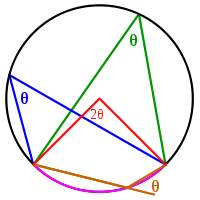Inscribed angle theorem

An inscribed angle (examples are the blue and green angles in the figure) is exactly half the corresponding central angle (red). Hence, all inscribed angles that subtend the same arc (pink) are equal. Angles inscribed on the arc (brown) are supplementary. In particular, every inscribed angle that subtends a diameter is a right angle (since the central angle is 180 degrees).

Sagitta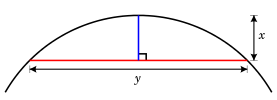The sagitta is the vertical segment.
• The sagitta (also known as the versine) is a line segment drawn perpendicular to a chord, between the midpoint of that chord and the arc of the circle.
• Given the length y of a chord, and the length x of the sagitta, the Pythagorean theorem can be used to calculate the radius of the unique circle which will fit around the two lines:Another proof of this result which relies only on two chord properties given above is as follows. Given a chord of length y and with sagitta of length x, since the sagitta intersects the midpoint of the chord, we know it is part of a diameter of the circle. Since the diameter is twice the radius, the "missing" part of the diameter is (2rx) in length. Using the fact that one part of one chord times the other part is equal to the same product taken along a chord intersecting the first chord, we find that (2rx)x = (y / 2)2. Solving for r, we find the required result.

Compass and straightedge constructions

There are many compass-and-straightedge constructions resulting in circles.

The simplest and most basic is the construction given the centre of the circle and a point on the circle. Place the fixed leg of the compass on the centre point, the movable leg on the point on the circle and rotate the compass.

Construct a circle with a given diameter

• Construct the midpoint M of the diameter.
• Construct the circle with centre M passing through one of the endpoints of the diameter (it will also pass through the other endpoint).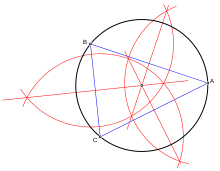Construct a circle through points A, B and C by finding the perpendicular bisectors (red) of the sides of the triangle (blue). Only two of the three bisectors are needed to find the centre.

Construct a circle through 3 noncollinear points

• Name the points P, Q and R,
• Construct the perpendicular bisector of the segment PQ.
• Construct the perpendicular bisector of the segment PR.
• Label the point of intersection of these two perpendicular bisectors M. (They meet because the points are not collinear).
• Construct the circle with centre M passing through one of the points P, Q or R (it will also pass through the other two points).

Circle of Apollonius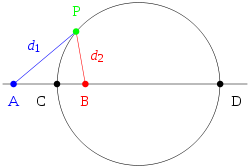Apollonius' definition of a circle: d1/d2 constant

Apollonius of Perga showed that a circle may also be defined as the set of points in a plane having a constant ratio (other than 1) of distances to two fixed foci, A and B. (The set of points where the distances are equal is the perpendicular bisector of A and B, a line.) That circle is sometimes said to be drawn about two points.

The proof is in two parts. First, one must prove that, given two foci A and B and a ratio of distances, any point P satisfying the ratio of distances must fall on a particular circle. Let C be another point, also satisfying the ratio and lying on segment AB. By the angle bisector theorem the line segment PC will bisect the interior angle APB, since the segments are similar:Analogously, a line segment PD through some point D on AB extended bisects the corresponding exterior angle BPQ where Q is on AP extended. Since the interior and exterior angles sum to 180 degrees, the angle CPD is exactly 90 degrees, i.e., a right angle. The set of points P such that angle CPD is a right angle forms a circle, of which CD is a diameter.

Second, see:p.15 for a proof that every point on the indicated circle satisfies the given ratio.

Cross-ratios

A closely related property of circles involves the geometry of the cross-ratio of points in the complex plane. If A, B, and C are as above, then the circle of Apollonius for these three points is the collection of points P for which the absolute value of the cross-ratio is equal to one:Stated another way, P is a point on the circle of Apollonius if and only if the cross-ratio [A,B;C,P] is on the unit circle in the complex plane.

Generalised circles

If C is the midpoint of the segment AB, then the collection of points P satisfying the Apollonius conditionis not a circle, but rather a line.

Thus, if A, B, and C are given distinct points in the plane, then the locus of points P satisfying the above equation is called a "generalised circle." It may either be a true circle or a line. In this sense a line is a generalised circle of infinite radius.

Circles inscribed in or circumscribed about other figures

In every triangle a unique circle, called the incircle, can be inscribed such that it is tangent to each of the three sides of the triangle.

About every triangle a unique circle, called the circumcircle, can be circumscribed such that it goes through each of the triangle's three vertices.

A tangential polygon, such as a tangential quadrilateral, is any convex polygon within which a circle can be inscribed that is tangent to each side of the polygon.

A cyclic polygon is any convex polygon about which a circle can be circumscribed, passing through each vertex. A well-studied example is the cyclic quadrilateral.

A hypocycloid is a curve that is inscribed in a given circle by tracing a fixed point on a smaller circle that rolls within and tangent to the given circle.

Circle as limiting case of other figures

The circle can be viewed as a limiting case of each of various other figures:

• A Cartesian oval is a set of points such that a weighted sum of the distances from any of its points to two fixed points (foci) is a constant. An ellipse is the case in which the weights are equal. A circle is an ellipse with an eccentricity of zero, meaning that the two foci coincide with each other as the centre of the circle. A circle is also a different special case of a Cartesian oval in which one of the weights is zero.
• A superellipse has an equation of the formfor positive a, b, and n. A supercircle has b = a. A circle is the special case of a supercircle in which n = 2.
• A Cassini oval is a set of points such that the product of the distances from any of its points to two fixed points is a constant. When the two fixed points coincide, a circle results.
• A curve of constant width is a figure whose width, defined as the perpendicular distance between two distinct parallel lines each intersecting its boundary in a single point, is the same regardless of the direction of those two parallel lines. The circle is the simplest example of this type of figure.

Squaring the circle

Squaring the circle is the problem, proposed by ancient geometers, of constructing a square with the same area as a given circle by using only a finite number of steps with compass and straightedge.

In 1882, the task was proven to be impossible, as a consequence of the Lindemann–Weierstrass theorem which proves that pi (π) is a transcendental number, rather than an algebraic irrational number; that is, it is not the root of any polynomial with rational coefficients.

Specially named circles

Of a torus

1. krikos, Henry George Liddell, Robert Scott, A Greek-English Lexicon, on Perseus
2. Arthur Koestler, The Sleepwalkers: A History of Man's Changing Vision of the Universe (1959)
3. Proclus, The Six Books of Proclus, the Platonic Successor, on the Theology of Plato Tr. Thomas Taylor (1816) Vol. 2, Ch. 2, "Of Plato"
4. Chronology for 30000 BC to 500 BC. History.mcs.st-andrews.ac.uk. Retrieved on 2012-05-03.
5. Squaring the circle. History.mcs.st-andrews.ac.uk. Retrieved on 2012-05-03.
6. Katz, Victor J. (1998), A History of Mathematics / An Introduction (2nd ed.), Addison Wesley Longman, p. 108, ISBN 978-0-321-01618-8
7. Posamentier and Salkind, Challenging Problems in Geometry, Dover, 2nd edition, 1996: pp. 104–105, #4–23.
8. College Mathematics Journal 29(4), September 1998, p. 331, problem 635.
9. Johnson, Roger A., Advanced Euclidean Geometry, Dover Publ., 2007.
10. Harkness, James (1898). Introduction to the theory of analytic functions. London, New York: Macmillan and Co. p. 30.
11. Ogilvy, C. Stanley, Excursions in Geometry, Dover, 1969, 14–17.
12. Altshiller-Court, Nathan, College Geometry, Dover, 2007 (orig. 1952).
13. Incircle – from Wolfram MathWorld. Mathworld.wolfram.com (2012-04-26). Retrieved on 2012-05-03.
14. Circumcircle – from Wolfram MathWorld. Mathworld.wolfram.com (2012-04-26). Retrieved on 2012-05-03.
15. Tangential Polygon – from Wolfram MathWorld. Mathworld.wolfram.com (2012-04-26). Retrieved on 2012-05-03.Wikimedia Commons has media related to: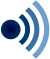Wikiquote has quotations related to: CirclesWikisource has the text of the 1911 Encyclopædia Britannica article Circle.